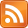Consider a matrix acting on a vector, $\mathbf{W}\mathbf{x}$. You can see this as function $f$ with type $\mathbb{R}^n \rightarrow \mathbb{R}^m$, where $\mathbf{W}$ is an $m \times n$ matrix, being applied to the vector $\mathbf{x}$. What is the derivative of $f$?

The framework behind taking derivatives in higher dimensions I covered in a previous post. I'll use the notation I introduced in that post here. $D(f)$ is the total derivative of a function $f$. $D(f)(\mathbf{x})$ is the total derivative of $f$ evaluated at a point $\mathbf{x}$. I'll also use the concept of a Jacobian matrix from that post.

I think the question "what is the derivative of a matrix?" is sort of funny and interesting in itself, but my motivation for exploring this came from machine learning — specifically, understanding the backpropagation algorithm we use to train neural networks. I'll show you what the derivative of a matrix is first, and then use this to justify a step in the backpropagation algorithm that had confused me.

Let's decide on some notation. Following Erik Learned-Miller I'm going to use $\frac{df}{d\mathbf{x}}$ to mean $D(f)(\mathbf{x})$. I'm not sure how standard this notation is, but I like it.

So, $f(\mathbf{x}) = \mathbf{W}\mathbf{x}$ and we want $\frac{df}{d \mathbf{x}}$. The derivative of a function $\mathbb{R}^n \rightarrow \mathbb{R}^m$ is represented by its $m \times n$ Jacobian matrix. Suppose $n = m = 2$. Then the Jacobian matrix of the function $f$ looks like this:

$$D(f) = \begin{bmatrix}\frac{\partial f_1}{\partial x_1} & \frac{\partial f_1}{\partial x_2}\\ & \\ \frac{\partial f_2}{\partial x_1} & \frac{\partial f_2}{\partial x_2}\end{bmatrix}$$

If we name the entries in our matrix and vector as follows:

$$\mathbf{W} = \begin{bmatrix}w_{11} & w_{12}\\ w_{21} & w_{22}\end{bmatrix}$$

$$\mathbf{x} = \begin{bmatrix}x_1\\ x_2\end{bmatrix}$$

then we can get those partial derivatives by first writing down what $f$ is as a function of two arguments:

\begin{aligned} f(x_1, x_2) &= \langle f_1(x_1, x_2), f_2(x_1, x_2) \rangle\\ f_1(x_1, x_2) &= x_1w_{11} + x_2w_{12}\\ f_2(x_1, x_2) &= x_1w_{21} + x_2w_{22}\\ \end{aligned}

and then computing:

\begin{aligned} \frac{\partial f_1}{\partial x_1} &= w_{11}\\ & \\ \frac{\partial f_1}{\partial x_2} &= w_{12}\\ & \\ \frac{\partial f_2}{\partial x_1} &= w_{21}\\ & \\ \frac{\partial f_2}{\partial x_2} &= w_{22}\\ \end{aligned}

giving us:

$$D(f) = \begin{bmatrix}\frac{\partial f_1}{\partial x_1} & \frac{\partial f_1}{\partial x_2}\\ & \\ \frac{\partial f_2}{\partial x_1} & \frac{\partial f_2}{\partial x_2}\end{bmatrix} = \begin{bmatrix}w_{11} & w_{12}\\ w_{21} & w_{22}\end{bmatrix} = \mathbf{W}$$

Conceptually, we can say $D(\mathbf{W}) = \mathbf{W}$.

So the derivative of a matrix is that matrix again. This makes sense because the total derivative of a function $f$ at a point $\mathbf{x}$ should tell us how a small vector with its tail at $\mathbf{x}$ is transformed by $f$. The total derivative is always a linear function on small vectors, regardless of whether $f$ is linear or not. But since in this case $f$ is already a linear function, the answer to "how does $f$ transform a small vector?" is the same as "how does $f$ transform any vector?"

Note we wrote the Jacobian as an $m \times n$ matrix. This seems good, because we want the Jacobian to represent a function on small vectors in the domain of the original function, $\mathbb{R}^n$, and an $m \times n$ matrix represents a function from $\mathbb{R}^n \rightarrow \mathbb{R}^m$.

However, sometimes you want the derivative of a function $\mathbb{R}^n \rightarrow \mathbb{R}$ to be a vector in $\mathbb{R}^n$. The problem is that it's the wrong shape; the Jacobian is a $1 \times n$ matrix, a row vector, but you want it to be an $n \times 1$ matrix, a column vector. So you need to take the transpose.

To illustrate: You have $\mathbf{d} = \mathbf{W}\mathbf{x}$ and $f(\mathbf{d})$ and you want to construct the column vector $\frac{df}{d\mathbf{x}}$. In neural networks, $f$ is a cost function evaluating your output, $\mathbf{d}$ is that output, $\mathbf{W}$ is a matrix of weights telling you how signals propagate from one layer of the network to the next layer, and $\mathbf{x}$ is your input data.

Apply the chain rule $D(f \circ g)(\mathbf{x}) = D(f)(g(\mathbf{x})) \circ D(g)(\mathbf{x})$ like this (we will identify the matrix $\mathbf{W}$ with the function it represents):

\begin{aligned} D(f)(\mathbf{x}) &= D(f)(\mathbf{W}\mathbf{x}) \circ D(\mathbf{W})(\mathbf{x})\\ D(f)(\mathbf{x}) &= D(f)(\mathbf{W}\mathbf{x}) \circ \mathbf{W}\\ D(f)(\mathbf{x}) &= D(f)(\mathbf{d}) \circ \mathbf{W}\\ \end{aligned}

where $D(\mathbf{W})(\mathbf{x}) = \mathbf{W}$ because the total derivative of a linear transformation is just that same transformation, and this is true at any point in the space. Now we can make a notational change, convert composition to matrix multiplication, and get:

\begin{aligned} \frac{df}{d\mathbf{x}} &= \frac{df}{d\mathbf{d}} \mathbf{W}\\ \end{aligned}

If $\mathbf{W}$ is an $m \times n$ matrix, $\frac{df}{d\mathbf{x}}$ is a row vector of shape $1 \times n$ and $\frac{df}{d\mathbf{d}}$ is a row vector of shape $1 \times m$. We want the left-hand side to have shape $n \times 1$ instead, so we take the transpose of both sides.

\begin{aligned} \left(\frac{df}{d\mathbf{x}}\right)^T &= \left(\frac{df}{d\mathbf{d}} \mathbf{W}\right)^T\\ & \\ \left(\frac{df}{d\mathbf{x}}\right)^T &= \mathbf{W}^T \left(\frac{df}{d\mathbf{d}}\right)^T\\ \end{aligned}

There's our formula for the derivative of $f$ at $\mathbf{x}$ as a column vector. In the context of reverse-mode automatic differentiation (the generalization of backpropagation), this tells you that if in "going forwards" you multiplied by a matrix, to "go backwards" you need to multiply by its transpose.

Resources I used in putting together this post: